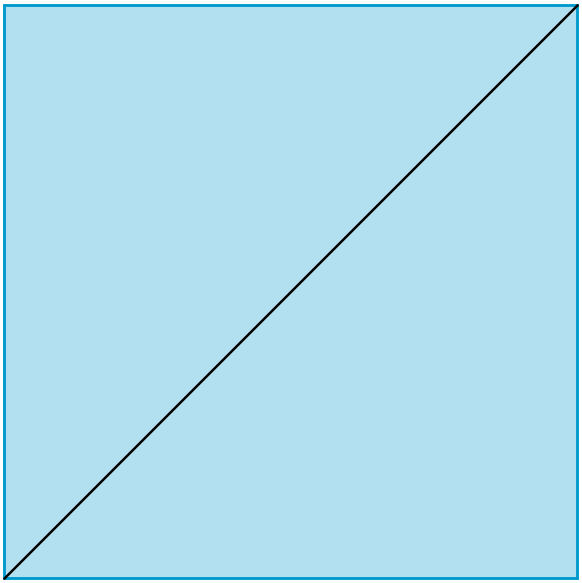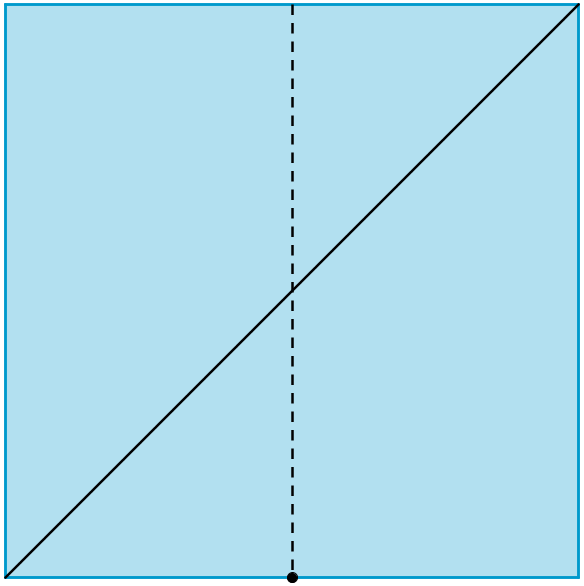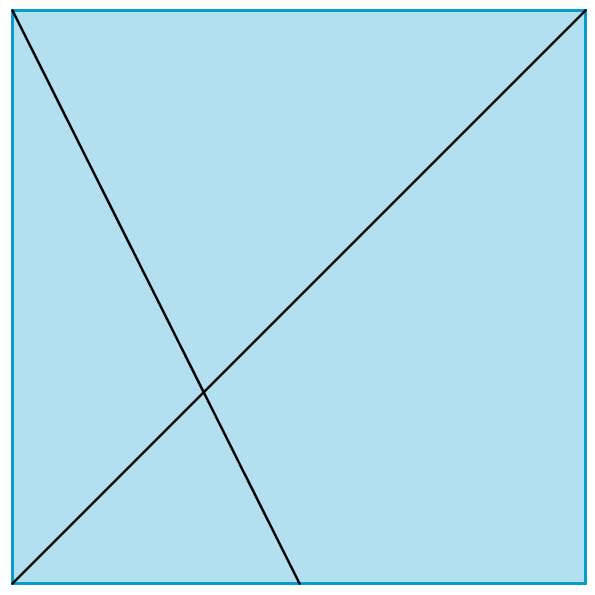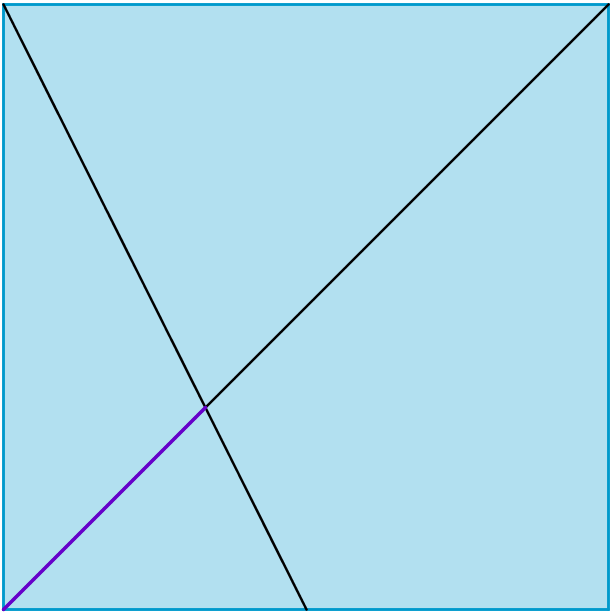#### You may also like### Just Opposite

A and C are the opposite vertices of a square ABCD, and have coordinates (a,b) and (c,d), respectively. What are the coordinates of the vertices B and D? What is the area of the square?### Fitting In

The largest square which fits into a circle is ABCD and EFGH is a square with G and H on the line CD and E and F on the circumference of the circle. Show that AB = 5EF. Similarly the largest equilateral triangle which fits into a circle is LMN and PQR is an equilateral triangle with P and Q on the line LM and R on the circumference of the circle. Show that LM = 3PQ### Pinned Squares

What is the total number of squares that can be made on a 5 by 5 geoboard?

# Folding Squares

##### Age 14 to 16 Challenge Level:##### What fractions of the diagonal do you think your new fold has created?

Measure the two sections of the diagonal and compare their lengths.
What do you notice?
Is this what you expected?

Can you produce a convincing mathematical argument or proof that justifies what you have found?

You might like to try A Parallelogram Trisection and Folding Fractions next.